# Find the volume of the solid bounded by the cylinder z2 + y2 =1, and the...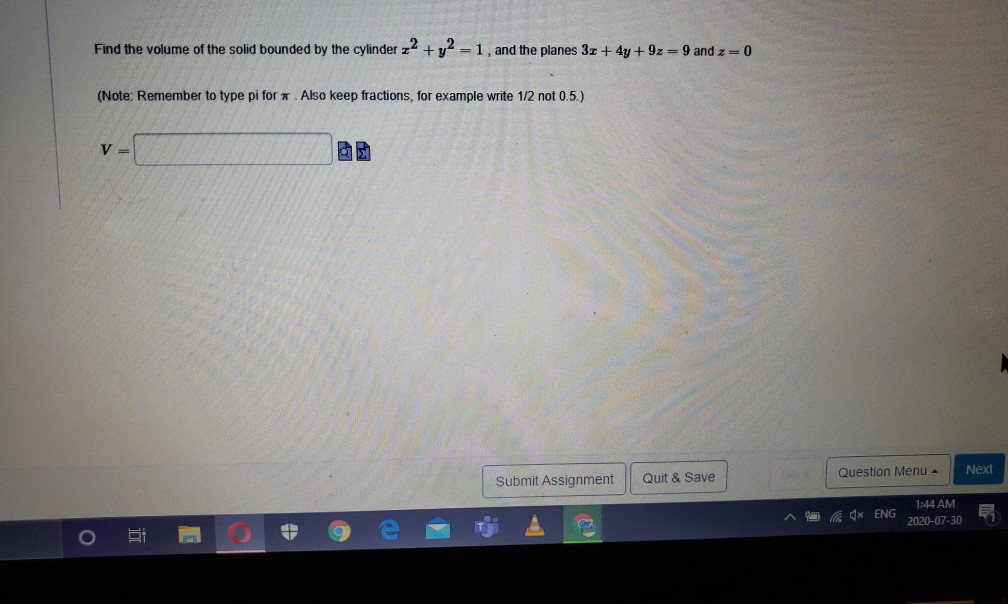Find the volume of the solid bounded by the cylinder z2 + y2 =1, and the planes 3 + 4y +9z=9 and z=0 (Note: Remember to type pi for. Also keep fractions, for example write 1/2 not 0.5.) V= Next Submit Assignment Quit & Save Question Menu 4x ENG 1:44 AM 2020-07-30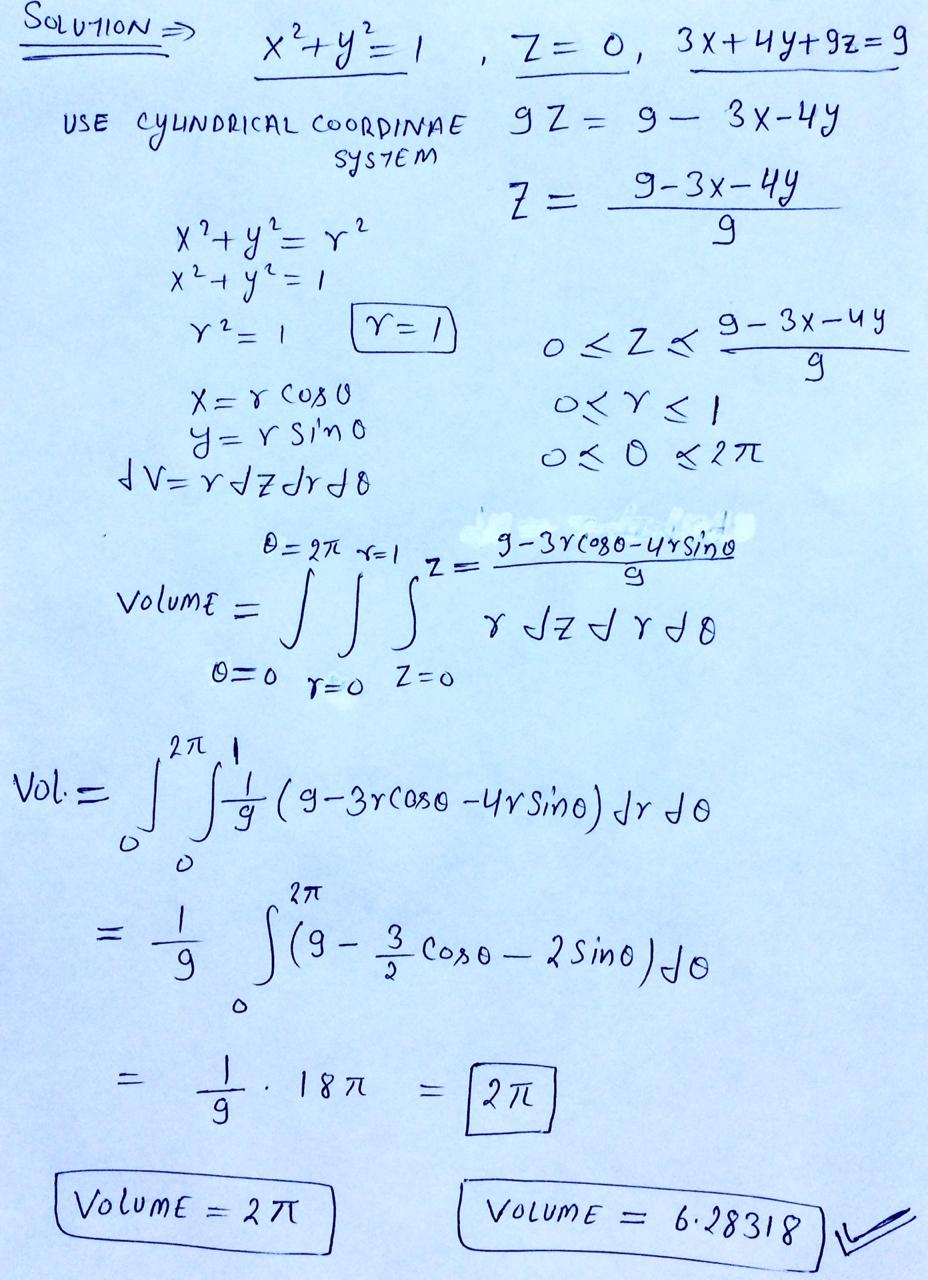V=2pi

##### Add Answer of: Find the volume of the solid bounded by the cylinder z2 + y2 =1, and the...
Similar Homework Help Questions
• ### Find the volume of the solid that lies inside the sphere 2 + y2 + 2...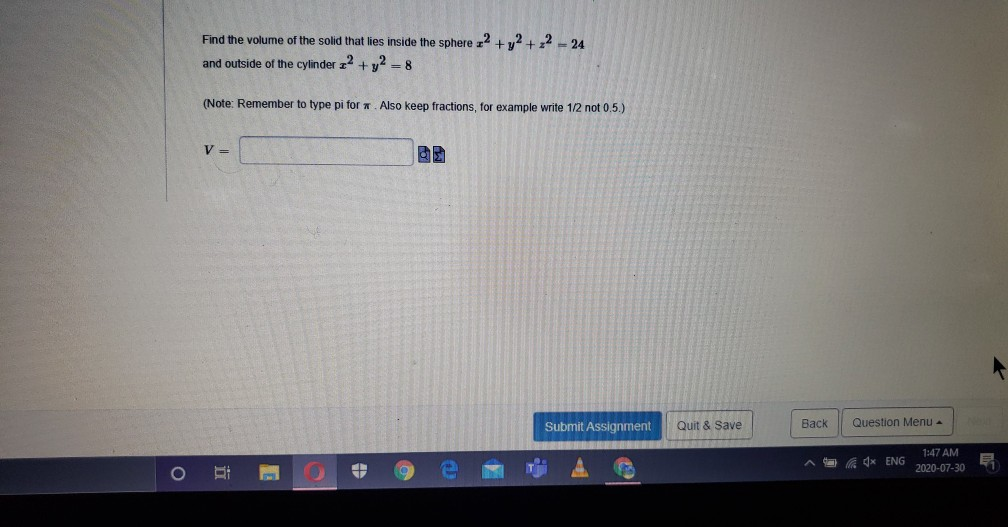Find the volume of the solid that lies inside the sphere 2 + y2 + 2 - 24 and outside of the cylinder 2 + y2 = 8 (Note: Remember to type pi for. Also keep fractions, for example write 1/2 not 0.5.) V Submit Assignment Quit & Save Back Question Menu - Ad* ENG 1:47 AM 2020-07-30

• ### Find the volume of the solid bounded by the cylinder x2 + y2 = 1, and...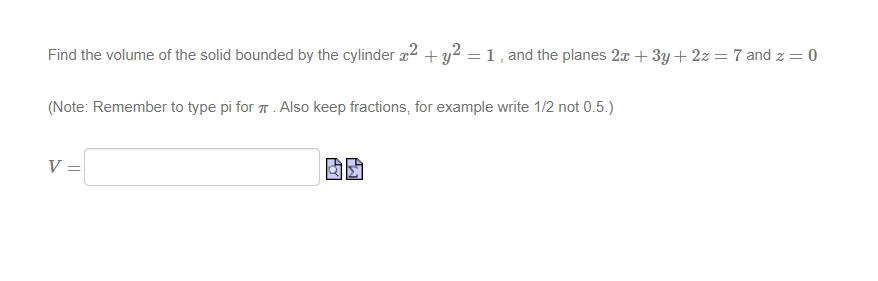Find the volume of the solid bounded by the cylinder x2 + y2 = 1, and the planes 2x + 3y + 2z = 7 and 2 = 0 (Note: Remember to type pi for 7. Also keep fractions, for example write 1/2 not 0.5.) V= M

• ### er 20 / Quiz 9 Remaining Time: 138:14 Evaluate 8 +y2 +22)-1/2 av where D is...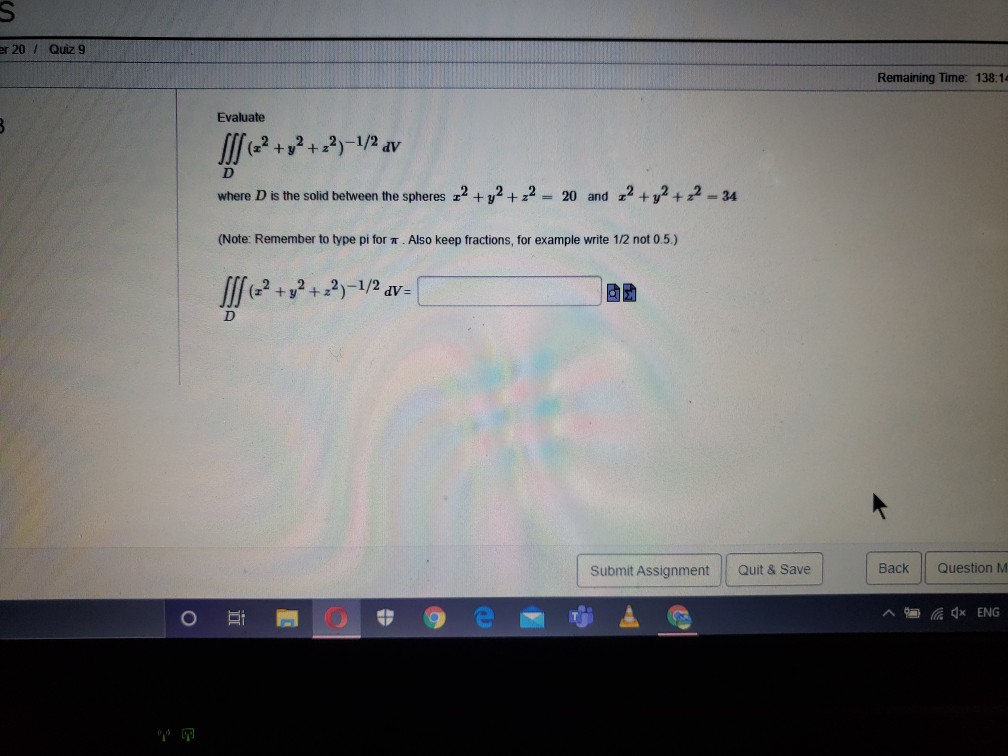er 20 / Quiz 9 Remaining Time: 138:14 Evaluate 8 +y2 +22)-1/2 av where D is the solid between the spheres 22 + y2 + 2 = 20 and 2 + y2 + 2 – 34 (Note: Remember to type pi for. Also keep fractions, for example write 1/2 not 0.5.) IS + y2 +22)-1/2 dv= D Submit Assignment Quit & Save Back Question M O 4x ENG

• ### Find the volume of the solid that lies inside the sphere x2 + y2 + 2...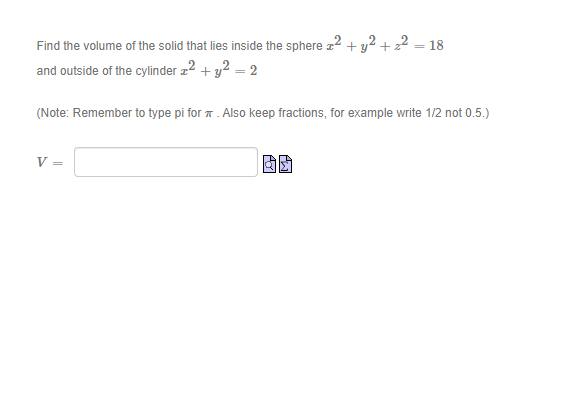Find the volume of the solid that lies inside the sphere x2 + y2 + 2 = 18 and outside of the cylinder 22 + y2 = 2 (Note: Remember to type pi for . Also keep fractions, for example write 1/2 not 0.5.) V=

• ### Evaluate Sl] (z2 + y2 + 22)-1/2 av je D where D is the solid between...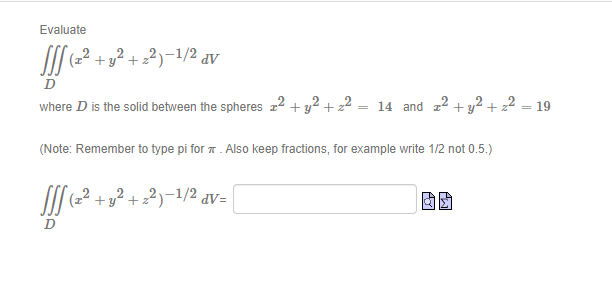Evaluate Sl] (z2 + y2 + 22)-1/2 av je D where D is the solid between the spheres 22 + y2 + 2 = 14 and 22 + y2 + 2 = 19 (Note: Remember to type pi for . Also keep fractions, for example write 1/2 not 0.5.) 11/ 62+72+27-14 + y2 + 22)-1/2 dv=

• ### Find the volume of the following solid regions. The solid bounded by the parabolic cylinder z...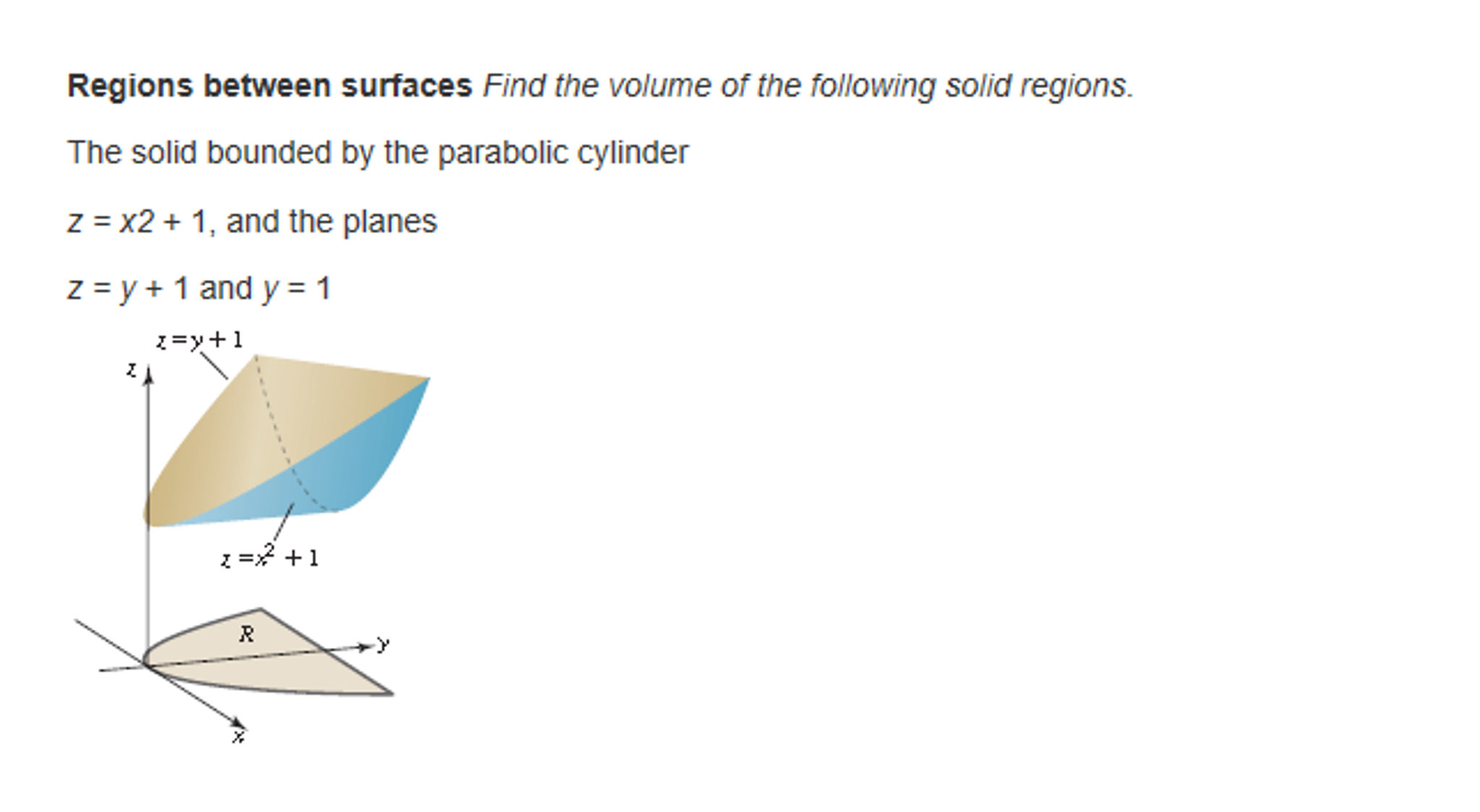Find the volume of the following solid regions. The solid bounded by the parabolic cylinder z = x2 +1, and the planes z = y + 1 and y = 1

• ### Find the volume of the following solid region: The solid bounded by the parabolic cylinder z...

Find the volume of the following solid region: The solid bounded by the parabolic cylinder z = x^2 +1, and the planes z = y+1 and y = 1

• ### Problem #1: Find the volume of the solid bounded by the graphs of x2 + y2...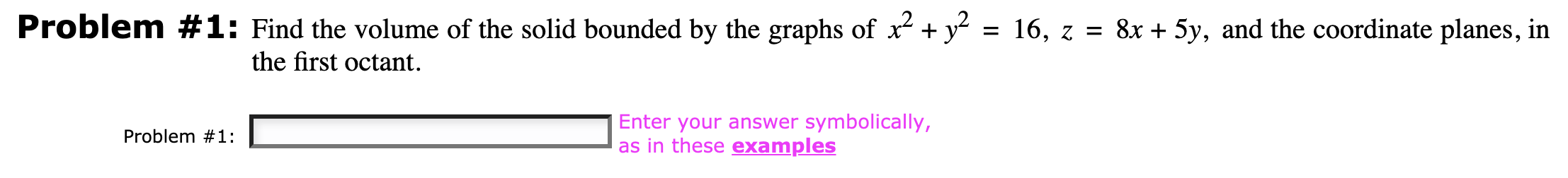Problem #1: Find the volume of the solid bounded by the graphs of x2 + y2 = 16, z = 8x + 5y, and the coordinate planes, in the first octant. Problem #1: Enter your answer symbolically, as in these examples

• ### Determine the total mass of a solid hemisphere bounded the surface x2 + y2 + z2-??...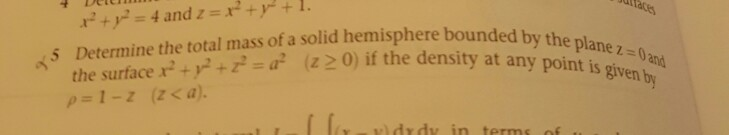Determine the total mass of a solid hemisphere bounded the surface x2 + y2 + z2-?? the plane 1 z-0 z0) if the density at any point is given b 0and 5 (z 20) if the densit

• ### 1) a.(20 pts) Set up the integral corresponding to the volume of the solid bounded above by the sphere x2+y2 + z2 16 and below by the cone z2 -3x2 + 3y2 and x 2 0 and y 20. You may want to graph...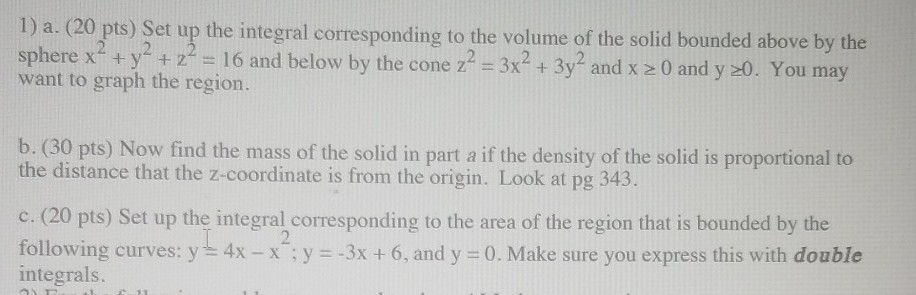1) a.(20 pts) Set up the integral corresponding to the volume of the solid bounded above by the sphere x2+y2 + z2 16 and below by the cone z2 -3x2 + 3y2 and x 2 0 and y 20. You may want to graph the region. b. (30 pts) Now find the mass of the solid in part a if the density of the solid is proportional to the distance that the z-coordinate is from the origin. Look at pg...

Free Homework App